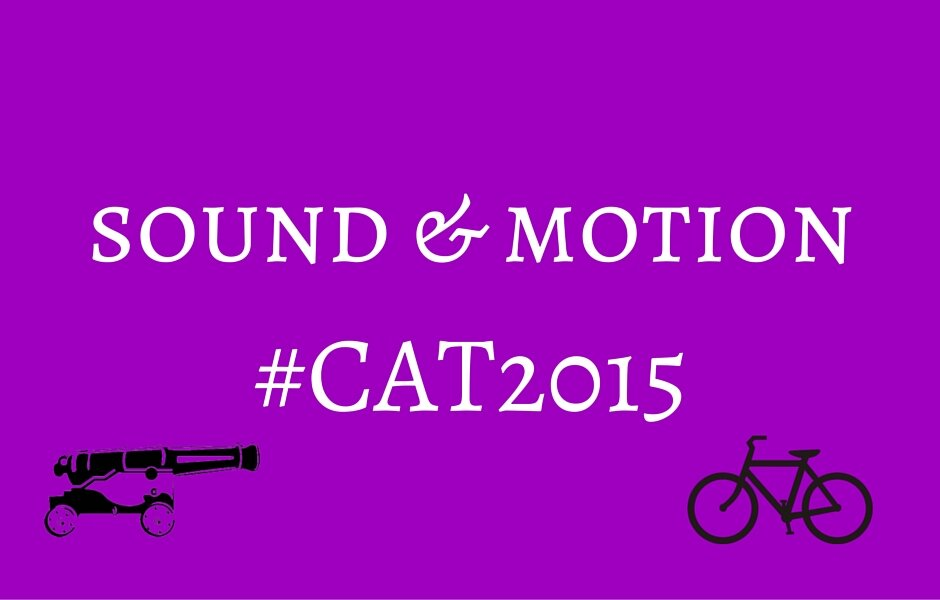Select PageA special type of questions from the topic of time, speed and distance is the one involving sound and motion. The question explains a scenario wherein, there is movement of sound from the source to the observer. In this article, I will try to make the concept a bit simpler than what it looks like.

The premise is that there are two sound waves emitted from a source. An observer is moving towards the source and encounters these sound waves one after the other. Because of the movement of both the sound wave and the observer in opposite directions, there is a gap between the two emissions and their observations. The question would either ask you to find the speed of sound or the speed at which the observer is moving.

Points to remember

(a) As with any other time, speed, distance question, the speed and the manner in which distance is covered is uniform

(b) There is no dampening of sound ie. it is to be assumed that the wave of sound will travel without any loss of intensity and would reach the observer as it is

(c) The speed of sound in air is around 343 m/s (it would be mentioned in the problem that has to be solved. In case you have to find the speed of sound and you do not have much time left to solve the question and desperately want to gamble, mark an answer which is in the 330 m/s to 345 m/s range. If there is more than one option that is in the 300-350 m/s range, better skip the question)

Let’s look at an example and see how the scenario pans out:

Two guns were fired from the same place at an interval of 10 minutes and 30 seconds, but a person in a train approaching the place hears second shot 10 minutes after the first. The speed of train (in km/hr), supposing that sound travels at 330 m/s is:

a) 19.8         b) 58.6          c) 59.4         d) 111.8

There are two ways to approach this question. Either you equate the distance traveled by the observer in 10 minutes to the distance traveled by sound in 30 seconds and get the answer to be 59.4 km/hr or you can calculate it using basic concepts of time, speed and distance when two objects are moving towards each other. The distance to be traveled will be the distance covered by the sound wave in 10.5 minutes ie. 630 seconds. This will be covered by the train and the sound wave together in 600 seconds which will give you the answer to be 59.4 m/s.

You can check out the video for the detailed solution to understand it in a better manner.

Edit: For the first method, we have to visualize that the distance covered would have been equivalent to that covered by sound in 10 minutes 30 seconds if it were not for the train moving towards it. What is indeed happening in this case is that both the entities are moving towards each other and because the train is moving for 10 minutes, sound has to move for 30 seconds lesser. In other words, if the train wasn’t moving for 10 minutes, it would have taken the sound wave 30 seconds to cover that distance. So, we say that the distance covered by sound in 30 seconds is equal to the distance covered by the train in 10 minutes.

Another example that you can try out:

Two shots are fired from a cannon at an interval of 35 seconds. A soldier who is approaching enemy territory by traveling underwater hears the two shots at a gap of 30 seconds. What is the speed of the sound in water if the soldier is traveling at a speed of 50 m/s towards the source?

(a) 300 m/s           (b) 330 m/s           (c) 360 m/s            (d) 375 m/s

We hope you have registered yourself for the CAT 2015 booster workshop series. The first workshop on Numbers will be on the coming Saturday.

error: Content is protected !!